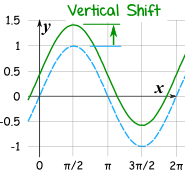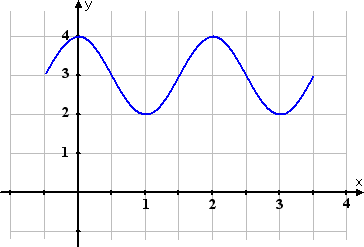# Properties of Trig Functions

About Trigonometric Functions: Trigonometric functions occur in any real world situation that continuously recurs after a certain point in time. Such real world examples could be the rise and fall between high and low tide, radio waves, electrical currents, the orbit of the earth, or even the spinning of a ferris wheel. These real world examples of sine and cosine functions are represented in a continuous graph that looks like waves. This occurs because of the recurrence of the functions periods. A period is the distance required for the function to complete one full cycle. For example, the time it takes for the highest point the tide reaches all the way to the lowest point of the tide and back to the highest point is one period. Another example is the time it takes for a ferris wheel to go around one time. Other properties are used as well to describe trigonometric functions such as the vertical shift of the function, the amplitude of the function, and phase shift of the function described below.

The graph of a trigonometric function is represented by:

Y= a +(-) b SIN/COS(cX +(-) d)

a=Vertical Shift
b=Amplitude
c=2π/period
d=(phase shift)/c

+b = no reflection
-b = reflection
+d= horizontal shift left
-d= horizontal shift right

#### Vertical Shift

The vertical shift moves the graph vertically, up or down. This movement is based on what happens to the y-value of the graph. The value of the vertical shift is the value of the graphs midline (center).#### Amplitude

The amplitude vertically stretches the function. the value of the amplitude in the function is the units from maximum point to the midline or minimum point to the midline.#### Period

The period is the distance required for the function to complete one full cycle.#### Phase Shift

Phase shift is the amount of horizontal displacement of the function from its original position.#### Reflection

The reflection of a trigonometric graph flips the graph over the x axis. This changes all the y values of the function. The reflection is represented by a negative on the amplitude in the trigonometric equation.### Transformations on Trig Functions### How to Graph Trig Functions

When Graphing Trig Functions like Sine there are 5 key point to know, so you have the curve right. The Starting Point (SP), the End Point (EP), the Middle Point (M), the Quarter 1 Point (Q1), and the Quarter Three Point (Q3). With these five points, and the knowledge of how a sinusoidal functions should look, you can graph a sinusoidal function. (Period is always represented by P)

(1)
\begin{equation} SP = Phase shift \end{equation}
(2)
\begin{equation} EP = SP+P \end{equation}
(3)
\begin{equation} M = (SP+EP)/2 \end{equation}
(4)
\begin{equation} Q1 = (SP+M)/2 \end{equation}
(5)
\begin{equation} Q3 = (M+EP)/2 \end{equation}
##### Key Trig Values for Graphing Without Transformations# Types of Trig Functions

## Sine#### As Degrees

Angle Sine of Angle Angle Sine of Angle
0 180° 0
30° 1/2 210° -1/2
45° √2/2 225° -√2/2
60° √3/2 240° 1√3/2
90° 1 270° -1
120° √3/2 300° -√3/2
135° √2/2 315° -√2/2
150° 1/2 330° -1/2

#### As Radians

Angle Sine of Angle Angle Sine of Angle
0 0 π 0
π/6 1/2 7π/6 -1/2
π/4 √2/2 5π/4 -√2/2
π/3 √3/2 4π/3 1√3/2
π/2 1 3π/2 -1
2π/3 √3/2 5π/3 -√3/2
3π/4 √2/2 7π/4 -√2/2
5π/6 1/2 -1/2

### Cosecant## Cosine### Secant## Tangent### Cotangent## Practice Problems

A.### Process

The amplitude of the graph is 2, so A = 2.

We know that one typical complete wave of the sine function starts at a y-value of 0, increases to 1, decreases through 0 and on to –1, and then increases to a y-value of 0, so the interval [-π/3, 3π +2π/3] contains one wave length of the function. The length of the interval tells us that the period is (3π +2π/3) - (-π/3) = 4π.

Using the formula: period = 2π/b , we set 4π=2π/b = and solve for B , finding that b=1/2.
We have already observed from the graph that the phase shift is -π/3, so we can use the formula:
phase shift = -c/b, set -π/3=c/(½) and solve for C , finding that c=π/6.

The equation of the graph is: $y=2sin((½)x+π/6)$

B.### Process

The amplitude of the graph is still 2, so A = 2.

We know that one typical complete wave of the cosine function starts at a y-value of 1, decreases through 0 to –1, and then increases through 0 to 1, so the interval [2 p /3, 4 p +2 p /3] contains one wave length of the function.

The length of the interval tells us that the period is 4 p +2 p /3 - 2 p /3 = 4 p , the same period as we found in Example 1, so b=½ as before.
We can use a phase shift of 2π/3, so the formula: phase shift = -c/b becomes 2π/3=-c/(½) and c= -π/3.

The equation of the graph is also: $y=2cos((½)x-π/3)$

c.

### Process

###### Now the phase shift. The phase shift = d/c. (π/2)/π=½